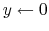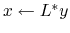Madagascar Programming Reference Manual## Implementation of operators

It should be evident by now that the implementation of an operatorshould have at least four arguments: a variable x from which the operand (entity on whichis applied)is read along with its length nx, and the variable y in which the resultis stored and its length.

Also, since every operator comes along with its adjoint, the implementation of the linear operators described later in this chapter, gives also the possibility to compute the adjoint operator. This is done through the boolean adj input argument. When adj is true, the adjoint operatorcomputed. As discussed before, the domains ofandare in general different, thereforecannot be applied on. However it can always be applied onor some, which has the same domain as. For this reason, when adj is true, the operand isand the result isand thus, y is used as input and the result is stored in x. As an example if [sec:sf_copy_lop]sf_copy_lop (the identity operator) is called, then the result is that. However if additionally adj is true, then the result will be. If [sec:adjnull]adjnull (the null operator) is called, then the result is that. However if additionally adj is true, then the result will be.

Finally, it is often the case that we need to computebut. For this reason another boolean argument, namely add is defined. If add is true, then. Considering the same example with the identity operator, if sf_copy_lop is called with add being true, then. If additionally adj is true, then. Or if adjnull is called with add being true, if adj is false,and if adj is true, then(so in essence, if add is true, no matter what the value of adj, nothing happens).

As a conclusion, the linear operators described in this chapter have all the following form:

 adj add description returns 0 0 normal operation0 1 normal operation with addition1 0 adjoint operation1 1 adjoint operation with additionMadagascar Programming Reference Manual